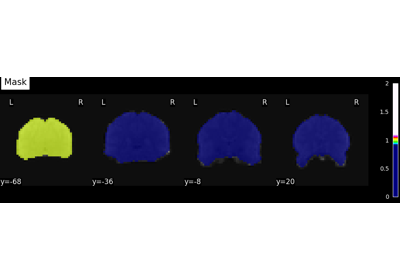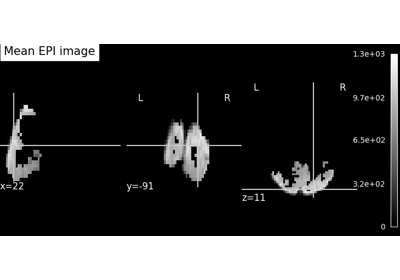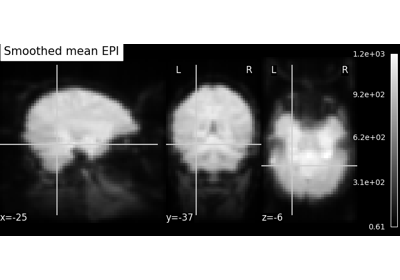Note

This page is a reference documentation. It only explains the function signature, and not how to use it. Please refer to the user guide for the big picture.

# nilearn.plotting.plot_roi#

nilearn.plotting.plot_roi(roi_img, bg_img=<MNI152Template>, cut_coords=None, output_file=None, display_mode='ortho', figure=None, axes=None, title=None, annotate=True, draw_cross=True, black_bg='auto', threshold=0.5, alpha=0.7, cmap=<matplotlib.colors.LinearSegmentedColormap object>, dim='auto', colorbar=False, cbar_tick_format='%i', vmin=None, vmax=None, resampling_interpolation='nearest', view_type='continuous', linewidths=2.5, **kwargs)[source]#

Plot cuts of an ROI/mask image (by default 3 cuts: Frontal, Axial, and Lateral)

Parameters:
roi_imgNiimg-like object

See Input and output: neuroimaging data representation. The ROI/mask image, it could be binary mask or an atlas or ROIs with integer values.

bg_imgNiimg-like object, optional

See Input and output: neuroimaging data representation. The background image to plot on top of. If nothing is specified, the MNI152 template will be used. To turn off background image, just pass “bg_img=None”. Default=MNI152TEMPLATE.

cut_coordsNone, a `tuple` of `float`, or `int`, optional

The MNI coordinates of the point where the cut is performed.

• If `display_mode` is ‘ortho’ or ‘tiled’, this should be a 3-tuple: `(x, y, z)`

• For `display_mode == 'x'`, ‘y’, or ‘z’, then these are the coordinates of each cut in the corresponding direction.

• If `None` is given, the cuts are calculated automatically.

• If `display_mode` is ‘mosaic’, and the number of cuts is the same for all directions, `cut_coords` can be specified as an integer. It can also be a length 3 tuple specifying the number of cuts for every direction if these are different.

Note

If `display_mode` is ‘x’, ‘y’ or ‘z’, `cut_coords` can be an integer, in which case it specifies the number of cuts to perform.

output_file`str`, or None, optional

The name of an image file to export the plot to. Valid extensions are .png, .pdf, .svg. If `output_file` is not None, the plot is saved to a file, and the display is closed.

display_mode{‘ortho’, ‘tiled’, ‘mosaic’,’x’,’y’, ‘z’, ‘yx’, ‘xz’, ‘yz’}, optional

Choose the direction of the cuts:

• ‘x’: sagittal

• ‘y’: coronal

• ‘z’: axial

• ‘ortho’: three cuts are performed in orthogonal directions

• ‘tiled’: three cuts are performed and arranged in a 2x2 grid

• ‘mosaic’: three cuts are performed along multiple rows and columns

Default=’ortho’.

figure`int`, or `matplotlib.figure.Figure`, or None, optional

Matplotlib figure used or its number. If `None` is given, a new figure is created.

axes`matplotlib.axes.Axes`, or 4 tupleof `float`: (xmin, ymin, width, height), optional

The axes, or the coordinates, in matplotlib figure space, of the axes used to display the plot. If `None`, the complete figure is used.

title`str`, or None, optional

The title displayed on the figure. Default=None.

annotate`bool`, optional

If `annotate` is `True`, positions and left/right annotation are added to the plot. Default=True.

draw_cross`bool`, optional

If `draw_cross` is `True`, a cross is drawn on the plot to indicate the cut position. Default=True.

black_bg`bool`, or ‘auto’, optional

If `True`, the background of the image is set to be black. If you wish to save figures with a black background, you will need to pass facecolor=’k’, edgecolor=’k’ to `matplotlib.pyplot.savefig`. Default=’auto’.

thresholda number, None, or ‘auto’, optional

If `None` is given, the image is not thresholded. If a number is given, it is used to threshold the image: values below the threshold (in absolute value) are plotted as transparent. If ‘auto’ is given, the threshold is determined magically by analysis of the image. Default=0.5.

alphafloat between 0 and 1, optional

Alpha sets the transparency of the color inside the filled contours. Default=0.7.

cmap`matplotlib.colors.Colormap`, or `str`, optional

The colormap to use. Either a string which is a name of a matplotlib colormap, or a matplotlib colormap object. Default=`plt.cm.gist_ncar`.

dim`float`, or ‘auto’, optional

Dimming factor applied to background image. By default, automatic heuristics are applied based upon the background image intensity. Accepted float values, where a typical span is between -2 and 2 (-2 = increase contrast; 2 = decrease contrast), but larger values can be used for a more pronounced effect. 0 means no dimming. Default=’auto’.

colorbarboolean, optional

If True, display a colorbar on the right of the plots. Default=False.

cbar_tick_format: str, optional

Controls how to format the tick labels of the colorbar. Ex: use “%.2g” to use scientific notation. Default is ‘%i’ to display as integers.

vmin`float`, optional

Lower bound of the colormap. If `None`, the min of the image is used. Passed to `matplotlib.pyplot.imshow`.

vmax`float`, optional

Upper bound of the colormap. If `None`, the max of the image is used. Passed to `matplotlib.pyplot.imshow`.

resampling_interpolation`str`, optional

Interpolation to use when resampling the image to the destination space. Can be:

• “continuous”: use 3rd-order spline interpolation

• “nearest”: use nearest-neighbor mapping.

Note

“nearest” is faster but can be noisier in some cases.

Default=’nearest’.

view_type{‘continuous’, ‘contours’}, optional

By default view_type == ‘continuous’, rois are shown as continuous colors. If view_type == ‘contours’, maps are shown as contours. For this type, label denoted as 0 is considered as background and not shown. Default=’continuous’.

linewidths`float`, optional

Set the boundary thickness of the contours. Only reflects when `view_type=contours`. Default=2.5.

`nilearn.plotting.plot_prob_atlas`

To simply plot probabilistic atlases (4D images)

Notes

A small threshold is applied by default to eliminate numerical background noise.

For visualization, non-finite values found in passed ‘roi_img’ or ‘bg_img’ are set to zero.

## Examples using `nilearn.plotting.plot_roi`#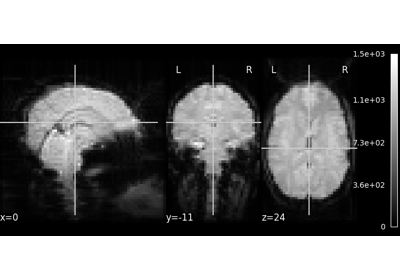A introduction tutorial to fMRI decoding

A introduction tutorial to fMRI decoding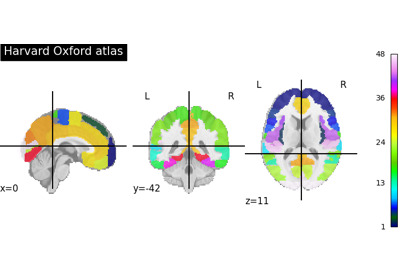Basic Atlas plotting

Basic Atlas plotting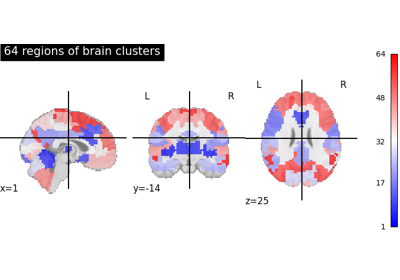Visualizing multiscale functional brain parcellations

Visualizing multiscale functional brain parcellations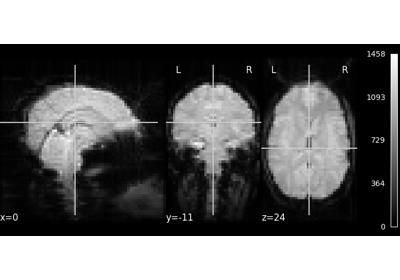NeuroImaging volumes visualization

NeuroImaging volumes visualization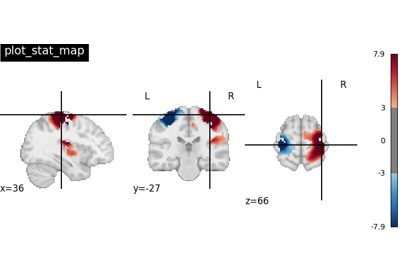Plotting tools in nilearn

Plotting tools in nilearn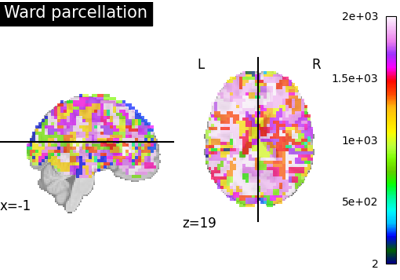Clustering methods to learn a brain parcellation from fMRI

Clustering methods to learn a brain parcellation from fMRIUnderstanding parameters of the first-level model

Understanding parameters of the first-level model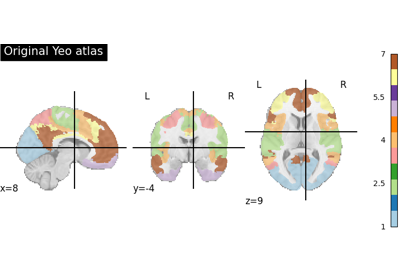Breaking an atlas of labels in separated regions

Breaking an atlas of labels in separated regions